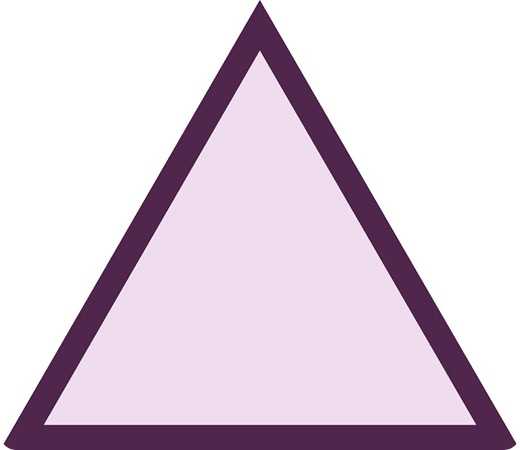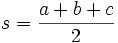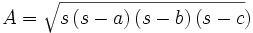How to find the area of a triangle

Want create site? Find Free WordPress Themes and plugins.

How to find the area of a triangle? Triangle is a shape just like the square, circle, oval, rectangle and etc. There are many kids of tringle such as isosceles triangle, right-angled triangle, equilateral triangle and etc. The one thing common is that they have three sides and three angles. Scalene has all sides of various length, isosceles has two sides to be the same with the other being different, so If you know one side you can know the other and an equilateral triangle is a basic one with all the sides of equal length. That means if you know that one side of the equilateral triangle is 5 cm and the other two sides are not given, you should know that they are also 5 cm. Triangles can be named after their angles. Where all the angles are less than 90 degrees makes it an acute triangle, with one angle as 90 degrees makes it a right angle, one angle more than 90 degrees but less 180 degrees makes it obtuse and when all angles have the same value, it is an equiangular triangle.

Related articles about How to find the area of a triangle

How to find the area of a circle

How to find the area of a trapezoid

How to find the mean

This is the formula for area of triangle. B is base and H is height of the figure. If you have a triangle, the lower part is known as the base and draw a straight line from the tip of the triangle to the bottom. That is the height. It is going to make 90 degrees with the base. If a triangle’s base and height is marked, it would look like there are two of them. One is a small right-angled triangle and the other is a big one.Calculation: Find out the area of a triangle whose height is 10 cm and base is 25 cm.

 Area = 1 bXh 2

Area =  x 25 cm X 10cm

= 125 cm²

Find out the height is the base is 10 cm and the area is 30 cm²

Area =  x b cm X b cm

30 = 0.5 x 10 x h

5h = 30

Height = 6 cm. (divide 30 by 5)

You can find out the area from its side using the Heron’s formulawhere s is half of the triangles perimeter, a, b and c are all the sides. S is needed for the formula of the area which isIf you know two sides and one angle, that is the angle between the known sides, you can use the formula that involves the sin. That means they could look like this Area = bc sin A. here b and c are going to be the sides you know and A is the angle.

Calculation: Find out the area of a triangle whose height two sides are 8 cm, 5 cm and the angle is 60 degrees.

Area = bc sin A

= 0.5 X 8 x5 x sin 60

= 20sin60 You can use the calculator to get the exact value and write the result in decimal places.

Did you find apk for android? You can find new Free Android Games and apps.

We will be happy to hear your thoughts

This site uses Akismet to reduce spam. Learn how your comment data is processed.